Courses

# Ex 9.1 NCERT Solutions(Part- 1)- Some Applications of Trigonometry Class 10 Notes | EduRev

## Class 10 : Ex 9.1 NCERT Solutions(Part- 1)- Some Applications of Trigonometry Class 10 Notes | EduRev

The document Ex 9.1 NCERT Solutions(Part- 1)- Some Applications of Trigonometry Class 10 Notes | EduRev is a part of the Class 10 Course Class 10 Mathematics by VP Classes.
All you need of Class 10 at this link: Class 10

Q.1. A circus artist is climbing a 20 m long rope, which is tightly stretched and tied from the top of a vertical pole to the ground. Find the height of the pole, if the angle made by the rope with the ground level is 30° (see figure).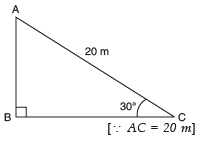Sol. Given: length of the rope (AC) = 20 m, ∠ACB = 30°
Let height of the pole (AB) = h metre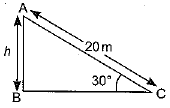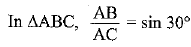⇒ h/20 = 1/2
⇒ h = 20/2 = 10 m
Hence, height of the pole = 10 m

Q.2. A tree breaks due to storm and the broken part bends so that the top of the tree touches the ground making an angle 30° with it. The distance between the foot of the tree to the point where the top touches the ground is 8 m. Find the height of the tree.
Sol. Let DB is a tree and AD is the broken part of it which touches the ground at C.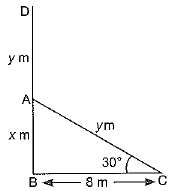Given: ∠ACB = 30º
and BC = 8m
Let AB = x m
∴ Now, length of the tree
= (x + y) m
In Δ ABC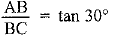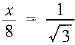⇒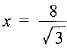... (i)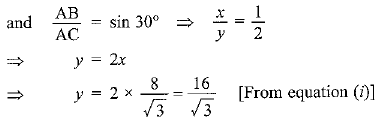Hence, total height of the tree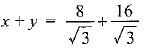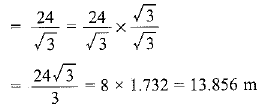Q3. A contractor plans to install two slides for the children to play in a park. For the children below the age of 5 years, she prefers to have a slide whose top is at a height of 1.5 m, and is inclined at an angle of 30° to the ground, whereas for older children, she wants to have a steep slide at a height of 3 m, and inclined at an angle of 60° to the ground. What should be the length of the slide in each case?
Sol. Let l1 is the length of slide for children below the age of 5 years and l2 is the length of the slide for elder children.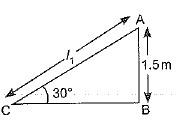In ΔABC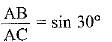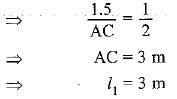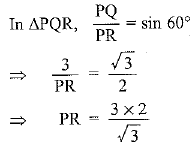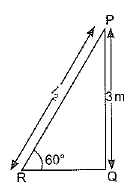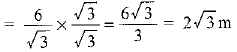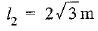Q.4. The angle of elevation of the top of a tower from a point on the ground, which is 30 m away from the foot of the tower, is 30°. Find the height of the tower.
Sol. Let h be the height of the tower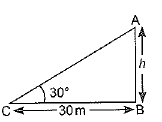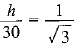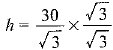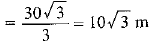Q.5. A kite is flying at a height of 60 m above the ground. The string attached to the kite is temporarily tied to a point on the ground. The inclination of the string with the ground is 60°. Find the length of the string, assuming that there is no slack in the string.
Sol. Given: height AB = 60 m, ∠ACB = 60°, AC = length of the string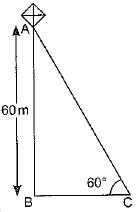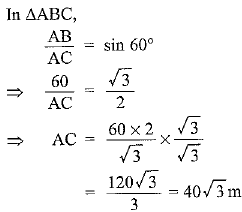Hence, length of the string = 40√3 m

Q.6. A 1.5 m tall boy is standing at some distance from a 30 m tall building. The angle of elevation from his eyes to the top of the building increases from 30° to 60° as he walks towards the building. Find the distance he walked towards the building.
Sol. Let  AB = height of the building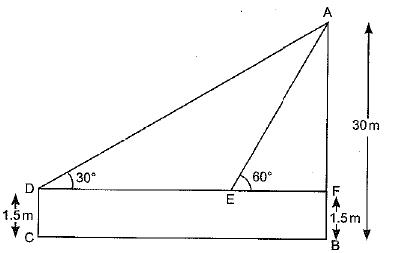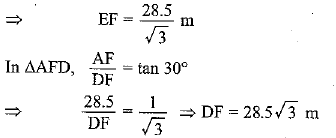The distance walked by the boy towards building
DE = DF - EF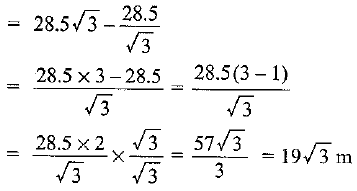Q.7. From a point on the ground, the angles of elevation of the bottom and the top of a transmission tower fixed at the top of a 20 m high building are 45° and 60° respectively. Find the height of the tower.
Sol. Given: AB = 20 m (Height of the building)
Let AD = h m (Height of the tower)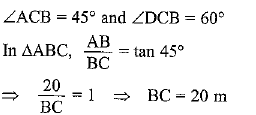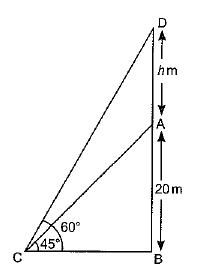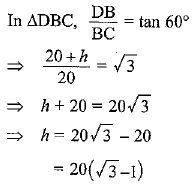Hence, height o f the tower =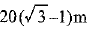Q.8. A statue, 1.6 m tall, stands on the top of a pedestal. From a point on the ground, the angle of elevation of the top of the statue is 60° and from the same point the angle of elevation of the top of the pedestal is 45°. Find the height of the pedestal.
Sol. Let the height of the pedestal AB = h m
Given: height of the statue = 1.6 m, ∠ACB = 45° and ∠DCB = 60°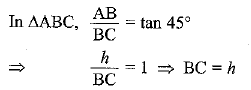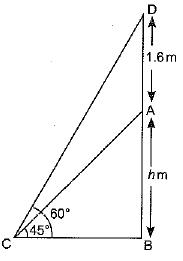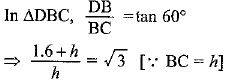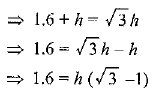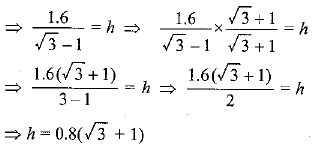Hence, height of the pedestal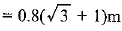Offer running on EduRev: Apply code STAYHOME200 to get INR 200 off on our premium plan EduRev Infinity!

132 docs

,

,

,

,

,

,

,

,

,

,

,

,

,

,

,

,

,

,

,

,

,

;